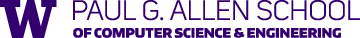# Randomized algorithms and probabilistic analysis#### Instructor: James R. Lee

Office hours: Tues, 3-4pm, CSE 640

#### Teaching assistant:

• Farzam Ebrahimnejad (febrahim@cs). Wed, 3-4pm, CSE2 223

#### Syllabus (approximate):

[Only a subset of topics are covered in a given offering.]
• Discrete probability
• The probabilistic method
• Linearity of expectation
• Variance and the 2nd moment method
• Lovasz Local Lemma
• Martingales
• Chernoff and Hoeffding-Azuma inequalities
• High-dimensional geometry and statistics
• Concentration of measure
• Dimension reduction
• Locality sensitive hashing
• Nearest-neighbor search
• Restricted isometry and compressive sensing
• Information and entropy
• Measures of information
• Max-entropy approximation
• Sparsification via sampling
• Multiplicative weights update and boosting
• Markov chains and convergence to equilibrium
• Random walks
• Eigenvalues and rapid mixing
• Expander graphs
• Markov chain Monte Carlo
• Approximate counting

## Lectures

#### [All lectures in a single file]

 Tue, Apr 02 First moments The probabilistic method Linearity of expectation The method of conditional expectation Markov’s inequality Crossing number inequalities Incidence geometry and sum-product estimates (Terry Tao’s blog) Thu, Apr 04 Second moments The $G_{n,p}$ model of random graphs Chebyshev’s inequality The second moment method Unbiased estimators Tue, Apr 09 More second moments Percolation on a tree Approximate counting for DNFs Random walk on the 2D torus Thu, Apr 11 Chernoff bounds Randomized rounding of LP solutions Chernoff bounds The method of Laplace transforms See this paper for the matching $\Omega(\frac{\log n}{\log \log n})$ integrality gap construction and an even stronger result, that the min-congestion disjoint-paths problem is NP-hard to apprixmate within any factor better than $O(\frac{\log n}{\log \log n})$. Concentration inequalities: A non-asymptotic theory of independence Tue, Apr 16 Applications of Chernoff bounds Balls in bins Randomized quicksort Negatively correlated random variables Survey on uniform spanning trees (Pemantle) Here you can find the proof of negative correlation Shayan’s class on spanning trees, electrical flows, and ATSP In the first eight lectures, you can find a discussion of the Thin Trees Conjecture, the use of large-deviation bounds for negatively dependent random variables, and a new approximation algorithm for the Asymmetric Traveling Salesman Problem (ATSP). Thu, Apr 18 Martingales and Azuma's inequality Martingales Doob martingales The vertex exposure martingale Azuma’s inequality Tue, Apr 23 Applications of martingales Proof of Azuma’s inequality Concentration of measure in product spaces Chromatic number of random graphs Thu, Apr 25 Random tree embeddings Embeddings and distortion Random partitions of metric spaces Memoryless random variables Tue, Apr 30 Bourgain's embedding Embeddings into Hilbert spaces Random zero-sets Thu, May 02 Dimensionality reduction Exponential moments for chi-squared random variables Laplace transform trick for independent sub-Gaussian entries Tue, May 07 Compressive sensing Sparse recovery The restricted isometry property $\ell_1$-minimization Thu, May 09 Sums of independent random matrices The method of exponential moments for symmetric matrices Golden-Thompson inequality (Terry Tao’s blog) Trace inequalities and quantum entropy Tue, May 14 Spectral sparsification Laplacians Effective resistance sampling Twice-Ramanjan Sparsifiers (Batson, Spielman, and Srivastava) Thu, May 16 Random walks and electrical networks Tue, May 21 Hitting times and cover times Thu, May 23 Markov chains and mixing times Improved Bounds for Randomly Sampling Colorings via Linear Programming (Chen, Delcourt, Moitra, Perarnau, and Postle) - The authors show that the Glauber dynamics on $k$-colorings is rapidly mixing for any $k \geq (11/6 - \epsilon_0) \Delta$, where $\Delta$ is the maximum degree and $\epsilon_0 > 0$ is some small constant. Tue, May 28 Markov chains and mixing times (cont.) Markov Chains and Mixing times (Levin-Peres-Wilmer) Thu, May 30 Eigenvalues, expansion, and rapid mixing Approximating the permanent (Jerrum and Sinclair) Tue, Jun 04 NO CLASS [final exam] Thu, Jun 06 NO CLASS [final exam]

## Homeworks

You may discuss problems with your classmates, but when you write down the solutions, you should do so by yourself. You can use the internet and books for reference material but you must cite every source that you consulted (the name of the book or web page suffices). You should also cite any classmates with whom you discussed solutions.

All homework should be turned in electronically to the Dropbox by the due date for the assignment. Homework should either be typeset (greatly preferred) or written very neatly and scanned.

Late HW policy: I will allow one (slightly) late homework per person (e.g., to accomodate for a paper deadline). This must be arranged beforehand.

Note on Bonus problems: These problems are usually quite challenging. If you do some of them, I will be impressed. But they are in no way required (or even suggested) for obtaining a good grade in the course.